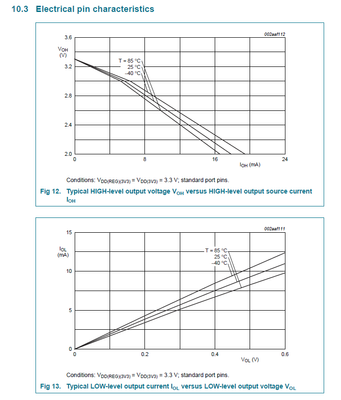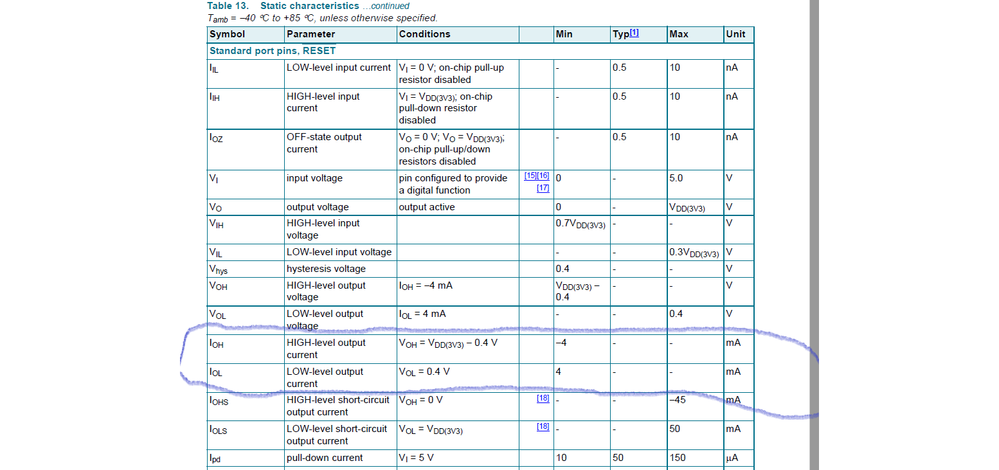## LPC1778FET208 GPIO Current Output

cancel
Showing results for
Did you mean:
SOLVED

## LPC1778FET208 GPIO Current Output

53 Views
Contributor I

Hi,

How much current we can draw from GPIO port pins in LPC1778FET208.

1 Solution
29 ViewsNXP TechSupport

Hi, Venkatesh,

The source current means the GPIO outputting current when the GPIO pin outputs logic high, the higher the source current is, the higher voltage drop is. The 4mA current means that we can guarantee that the voltage drop is less than 400mV when the source current is 4mA.

I copy the Fig from the data sheet of LPC177x. which can be downloaded from the link:

https://www.nxp.com.cn/docs/en/data-sheet/LPC178X_7X.pdf

The sink current is the current the pin draws when the GPIO pin outputs LOW logic. The higher the sink current is, the higher the GPIO pin voltage is. The 4mA sink current means that we can guarantee the pin voltage rising is less than 400mV when the pin draws 4mA current.3 Replies
42 ViewsNXP TechSupport

Hi, Friend,

Pls refer to Table 13. Static characteristics … in data sheet of LPC177x, both the source/sink current is 4mA.BR

XiangJun Rong

38 Views
Contributor I

Hi Xiangjun,

Thanks for your kind reply, in datasheet it is mentioned only minimum current this means we can able to draw 4mA maximum.

30 ViewsNXP TechSupport

Hi, Venkatesh,

The source current means the GPIO outputting current when the GPIO pin outputs logic high, the higher the source current is, the higher voltage drop is. The 4mA current means that we can guarantee that the voltage drop is less than 400mV when the source current is 4mA.

I copy the Fig from the data sheet of LPC177x. which can be downloaded from the link:

https://www.nxp.com.cn/docs/en/data-sheet/LPC178X_7X.pdf

The sink current is the current the pin draws when the GPIO pin outputs LOW logic. The higher the sink current is, the higher the GPIO pin voltage is. The 4mA sink current means that we can guarantee the pin voltage rising is less than 400mV when the pin draws 4mA current.